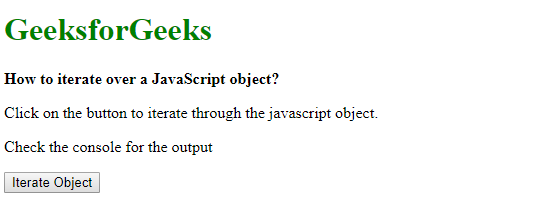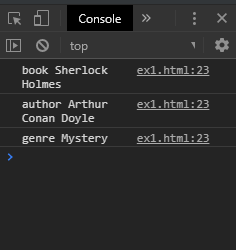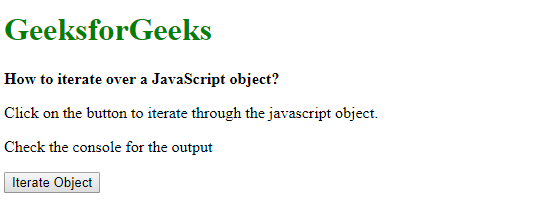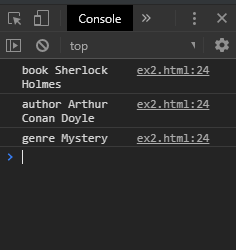# How to iterate over a JavaScript object ?

There are two methods to iterate over an object which are discussed below:

Method 1: Using for…in loop: The properties of the object can be iterated over using a for..in loop. This loop is used to iterate over all non-Symbol iterable properties of an object. Some objects may contain properties that may be inherited from their prototypes. The hasOwnProperty() method can be used to check if the property belongs to the object itself. The value of each key of the object can be found by using the key as the index of the object.

Syntax:

 `for` `(let key ``in` `exampleObj) { ` `    ``if` `(exampleObj.hasOwnProperty(key)) { ` `        ``value = exampleObj[key]; ` `        ``console.log(key, value); ` `    ``} ` `} `

Example:

 ` ` `<``html``> ` ` `  `<``head``> ` `    ``<``title``> ` `        ``How to iterate over ` `        ``a JavaScript object? ` `    `` ` ` ` ` `  `<``body``> ` `    ``<``h1` `style``=``"color: green"``> ` `        ``GeeksforGeeks ` `    `` ` `     `  `    ``<``b``> ` `        ``How to iterate over ` `        ``a JavaScript object?  ` `    `` ` `     `  `    ``<``p``> ` `        ``Click on the button to iterate ` `        ``through the javascript object. ` `    `` ` `     `  `    ``<``p``> ` `        ``Check the console ` `        ``for the output ` `    `` ` `     `  `    ``<``button` `onclick``=``"iterateObject()"``> ` `        ``Iterate Object ` `    `` ` `     `  `    ``<``script` `type``=``"text/javascript"``> ` `        ``function iterateObject() { ` `            ``let exampleObj = { ` `                ``book: "Sherlock Holmes", ` `                ``author: "Arthur Conan Doyle", ` `                ``genre: "Mystery" ` `            ``}; ` `     `  `            ``for (let key in exampleObj) { ` `                ``if (exampleObj.hasOwnProperty(key)) ` `                ``{ ` `                    ``value = exampleObj[key]; ` `                    ``console.log(key, value); ` `                ``} ` `            ``} ` `        ``} ` `    `` ` ` ` ` `  ` `

Output:

• Before clicking the button:• After clicking the button:Method 2: Object.entries() map: The Object.entries() method is used to return an array of the object’s own enumerable string-keyed property pairs. The returned array is used with the map() method to extract the key and value from the pairs.
The key and values from the key-value pair can be extracted by accessing the first and second index of the array pair. The first index corresponds to the key and the second index corresponds to the value of the pair.

Syntax:

 `Object.entries(exampleObj).map(entry => { ` `    ``let key = entry; ` `    ``let value = entry; ` `    ``console.log(key, value); ` `}); `

Example:

 ` ` `<``html``> ` `     `  `<``head``> ` `    ``<``title``> ` `        ``How to iterate over  ` `        ``a JavaScript object? ` `    `` ` ` ` ` `  `<``body``> ` `    ``<``h1` `style``=``"color: green"``> ` `        ``GeeksforGeeks ` `    `` ` `     `  `    ``<``b``> ` `        ``How to iterate over ` `        ``a JavaScript object?  ` `    `` ` `     `  `    ``<``p``> ` `        ``Click on the button to iterate ` `        ``through the javascript object. ` `    `` ` `     `  `    ``<``p``> ` `        ``Check the console ` `        ``for the output ` `    `` ` `     `  `    ``<``button` `onclick``=``"iterateObject()"``> ` `        ``Iterate Object ` `    `` ` `     `  `    ``<``script` `type``=``"text/javascript"``> ` `        ``function iterateObject() { ` `            ``let exampleObj = { ` `                ``book: "Sherlock Holmes", ` `                ``author: "Arthur Conan Doyle", ` `                ``genre: "Mystery" ` `            ``}; ` `     `  `            ``Object.entries(exampleObj).map(entry => { ` `                ``let key = entry; ` `                ``let value = entry; ` `                ``console.log(key, value); ` `            ``}); ` `        ``} ` `    `` ` ` ` ` `  ` `

Output:

• Before clicking the button:• After clicking the button:My Personal Notes arrow_drop_upCheck out this Author's contributed articles.

If you like GeeksforGeeks and would like to contribute, you can also write an article using contribute.geeksforgeeks.org or mail your article to contribute@geeksforgeeks.org. See your article appearing on the GeeksforGeeks main page and help other Geeks.

Please Improve this article if you find anything incorrect by clicking on the "Improve Article" button below.